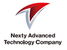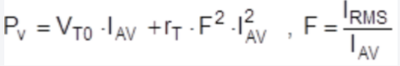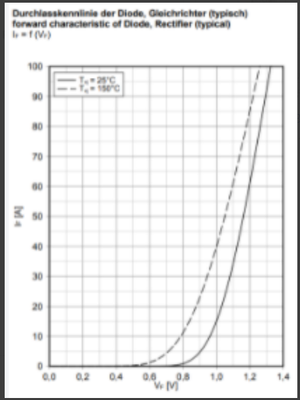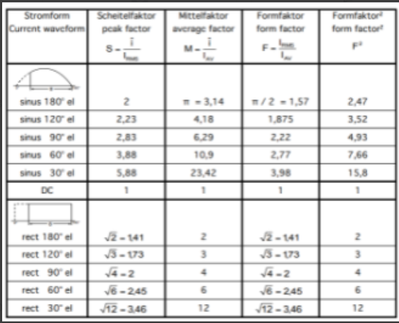# IGBT Forum DiscussionsLevel 3Level 3

# Converter Power Loss

Dear all,

Regarding FP50R12N2T4 and FP75R12N2T4,
do you have Converter Power Loss Curve , which is the “ AVERAGE FORWARD POWER DISSIPATION “ of attached file ?

BR
1 SolutionModeratorModerator

# Re: Converter Power Loss

Hi,

Calculating the power loss curve is not possible because it is highly dependent on current waveform seen during operation.

Therefore, as a first step, we would have to do the calculation manually and with a theoretical approach.

For power loss calculation on the rectifier side (per diode), please use the following formula.*Iav(per diode)=0.33*Idc

*Idc --> From customer operation

*F --> depending on the current waveform. For B6 configuration (our case), and if we assume ideal induction loads we can consider a Form Factor of 1.73.Regards,

Abhilash PModeratorModerator

# Re: Converter Power Loss

Hi,

Calculating the power loss curve is not possible because it is highly dependent on current waveform seen during operation.

Therefore, as a first step, we would have to do the calculation manually and with a theoretical approach.

For power loss calculation on the rectifier side (per diode), please use the following formula.*Iav(per diode)=0.33*Idc

*Idc --> From customer operation

*F --> depending on the current waveform. For B6 configuration (our case), and if we assume ideal induction loads we can consider a Form Factor of 1.73.Regards,

Abhilash P